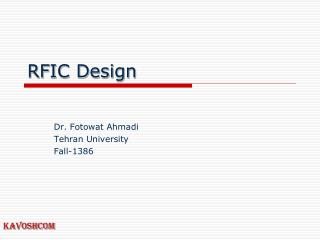# RFIC Design - PowerPoint PPT PresentationDownload PresentationRFIC Design

RFIC DesignDownload Presentation## RFIC Design

- - - - - - - - - - - - - - - - - - - - - - - - - - - E N D - - - - - - - - - - - - - - - - - - - - - - - - - - -
##### Presentation Transcript

1. RFIC Design Dr. Fotowat Ahmadi Tehran University Fall-1386

2. RFIC Design Section Zero

3. Section Zero

4. Section Zero

5. Section Zero

6. Section Zero

7. Section Zero

8. Section Zero

9. Section Zero

10. Section Zero

11. Section Zero

12. Section Zero

13. Section Zero

14. Section Zero

15. Section Zero

16. Section Zero Impedance Ideal capacitor capacitive inductive frequency Fr

17. Section Zero

18. Section Zero

19. 18’

20. Section Zero

21. Section Zero

22. Section Zero

23. Section Zero

24. Section Zero

25. Section Zero

26. Section Zero

27. Section Zero

28. Section Zero

29. Section Zero

30. Section Zero

31. Section Zero

32. Section Zero

33. Section Zero

34. Section Zero

35. Section Zero 12.87pf 384nH 12.87pf 87nH 87nH

36. Section Zero

37. Section Zero

38. Section Zero

39. Section Zero

40. Section Zero

41. Section Zero

42. Section Zero

43. Section Zero

44. Section Zero

45. Section Zero

46. Section Zero

47. Section Zero What if an impedance match is required over fairly wide bandwidth? Select a virtual resistor to be in between the source and load. 46

48. Section Zero

49. Section Zero